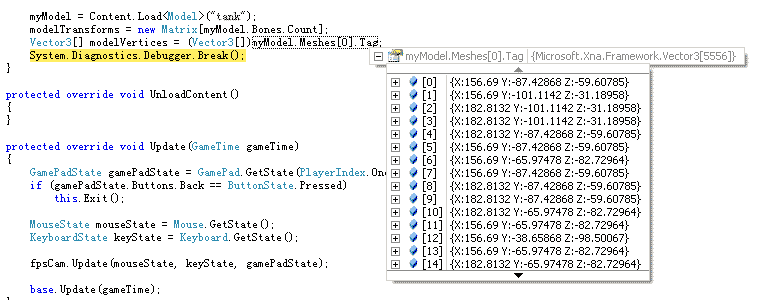# 4.14 通过扩展模型处理器直接处理每个ModelMesh的顶点位置数据

## 解决方案

public override ModelContent Process(NodeContent input,ContentProcessorContext context)
{
List<Vector3[]> modelVertices = new List<Vector3[]>();
} 

private List<Vector3[]> AddModelMeshVertexArrayToList(NodeContent node, List<Vector3[]>modelVertices)
{
foreach (NodeContent child in node.Children)
MeshContent mesh = node as MeshContent;
if (mesh != null)
{
List<Vector3> nodeVertices = new List<Vector3>();
foreach (GeometryContent geo in mesh.Geometry)
{
foreach (int index in geo.Indices)
{
Vector3 vertex = geo.Vertices.Positions[index];
}
}
}
return modelVertices;
}

ModelContent usualModel = base.Process(input, context);
int i = 0;
foreach(ModelMeshContent mesh in usualModel.Meshes)
mesh.Tag = modelVertices[i++];
return usualModel; 

ModelContent usualModel = base.Process(input, context);
int i = 0;
foreach (ModelMeshContent mesh in usualModel.Meshes)
{
List<Vector3> modelMeshVertices = new List<Vector3>();
foreach (ModelMeshPartContent part in mesh.MeshParts)
{
}
mesh.Tag = modelMeshVertices.ToArray();
}
return usualModel; 

myModel = Content.Load<Model>("tank");
modelTransforms = new Matrix[myModel.Bones.Count]; 

Vector3[] modelVertices = (Vector3[])myModel.Meshes.Tag;
System.Diagnostics.Debugger.Break(); 

## 代码

namespace Vector3Pipeline
{
[ContentProcessor]
public class ModelVector3Processor : ModelProcessor
{
public override ModelContent Process(NodeContent input, ContentProcessorContext context)
{
List<Vector3[]> modelVertices = new List<Vector3[]>();

ModelContent usualModel = base.Process(input, context);
int i = 0;
foreach (ModelMeshContent mesh in usualModel.Meshes)
{
List<Vector3> modelMeshVertices = new List<Vector3>();
foreach (ModelMeshPartContent part in mesh.MeshParts)
{
}
mesh.Tag = modelMeshVertices.ToArray();
}
return usualModel;
}

private List<Vector3[]> AddModelMeshVertexArrayToList(NodeContent node, List<Vector3[]> modelVertices)
{
foreach (NodeContent child in node.Children)
MeshContent mesh = node as MeshContent;
if (mesh != null)
{
List<Vector3> nodeVertices = new List<Vector3>();
foreach (GeometryContent geo in mesh.Geometry)
{
foreach (int index in geo.Indices)
{
Vector3 vertex = geo.Vertices.Positions[index];
}
}
}